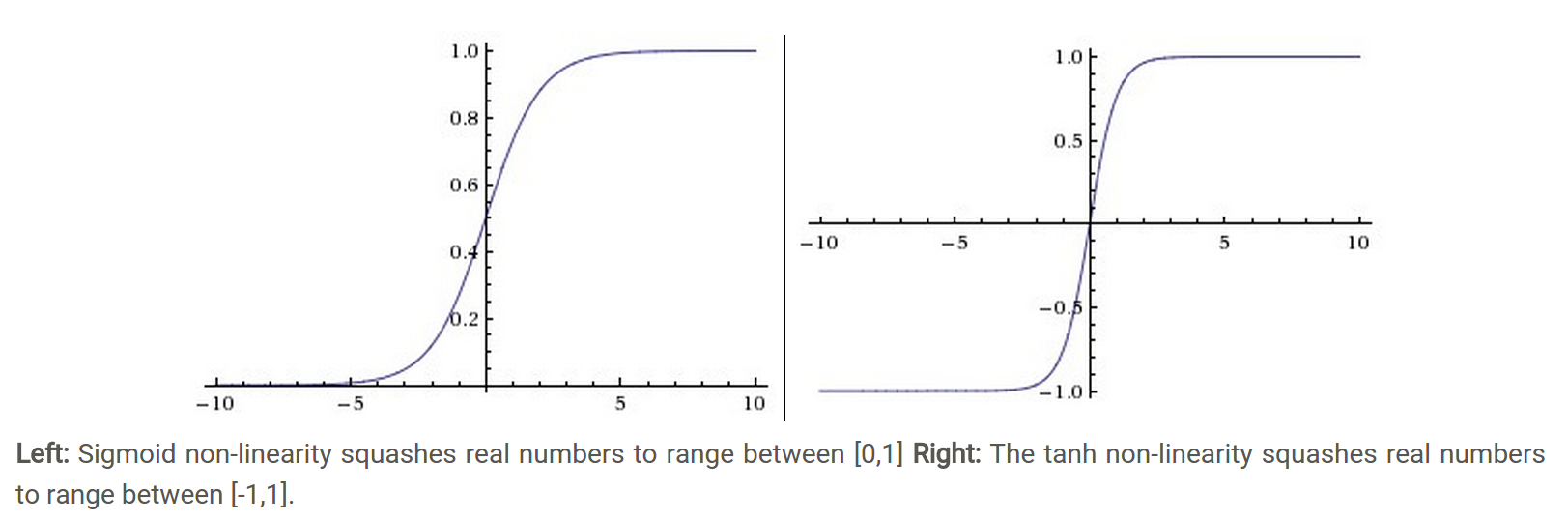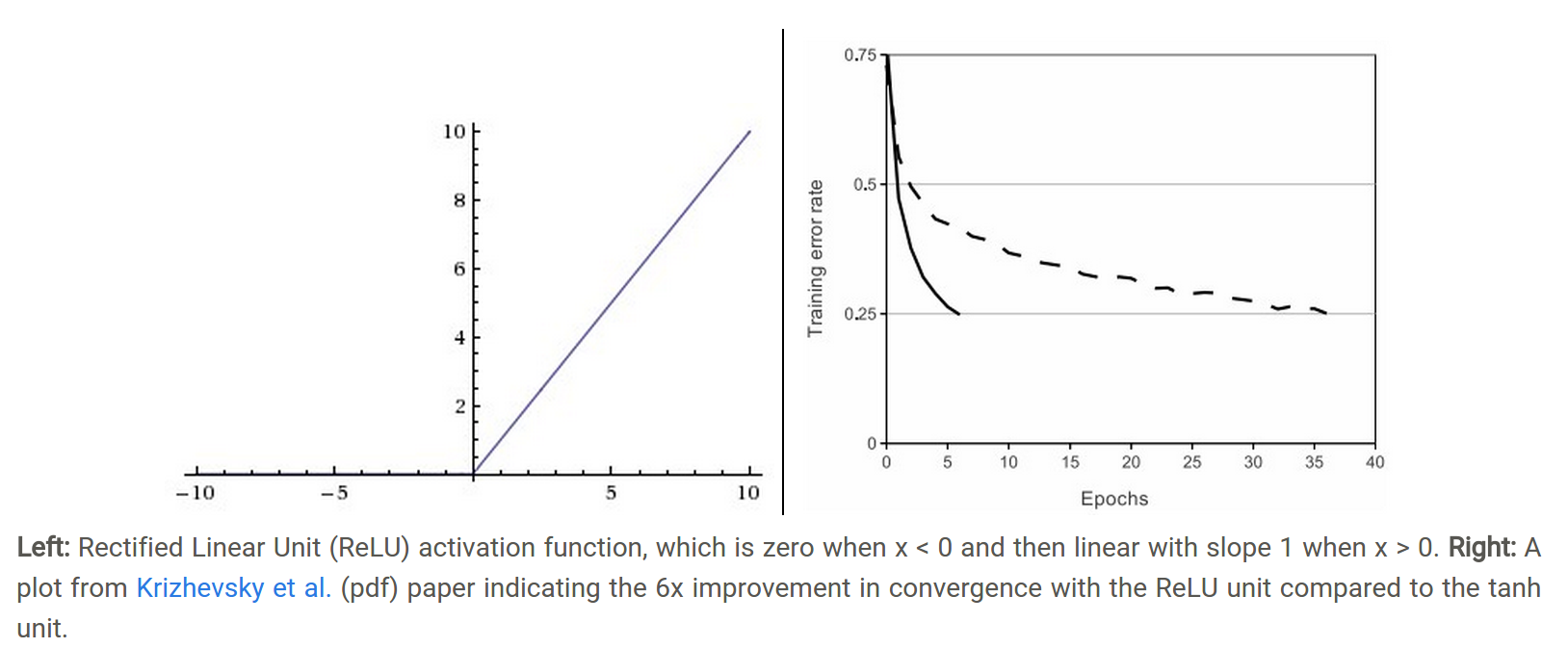# Deep learning系列（七）激活函数

### 1. sigmoid激活函数

sigmoid将一个实数输入映射到[0,1]范围内，如下图（左）所示。使用sigmoid作为激活函数存在以下几个问题：

• 梯度饱和。当函数激活值接近于0或者1时，函数的梯度接近于0。在反向传播计算梯度过程中：δ(l)=(W(l))Tδ(l+1)f(z(L))$\delta^{(l)}=(W^{(l)})^T\delta^{(l+1)}*f'(z^{(L)})$，每层残差接近于0，计算出的梯度也不可避免地接近于0。这样在参数微调过程中，会引起参数弥散问题，传到前几层的梯度已经非常靠近0了，参数几乎不会再更新。
• 函数输出不是以0为中心的。我们更偏向于当激活函数的输入是0时，输出也是0的函数。### 2. tanh激活函数

tanh函数将一个实数输入映射到[-1,1]范围内，如上图（右）所示。当输入为0时，tanh函数输出为0，符合我们对激活函数的要求。然而，tanh函数也存在梯度饱和问题，导致训练效率低下。

### 3.Relu激活函数

Relu激活函数（The Rectified Linear Unit）表达式为：f(x)=max(0,x)$f(x)=max(0,x)$。如下图（左）所示：• 梯度不饱和。梯度计算公式为：1{x>0}$1\{x>0\}$。因此在反向传播过程中，减轻了梯度弥散的问题，神经网络前几层的参数也可以很快的更新。
• 计算速度快。正向传播过程中，sigmoid和tanh函数计算激活值时需要计算指数，而Relu函数仅需要设置阈值。如果x<0,f(x)=0$x<0, f(x)=0$，如果x>0,f(x)=x$x>0, f(x) = x$。加快了正向传播的计算速度。

1. http://cs231n.stanford.edu/syllabus.html
2. Krizhevsky A, Sutskever I, Hinton G E. Imagenet classification with deep convolutional neural networks. NIPS. 2012: 1097-1105.

©️2019 CSDN 皮肤主题: 大白 设计师: CSDN官方博客